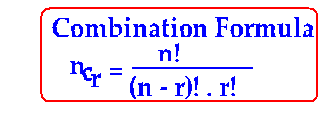## Binomial Theorem - Introduction

Click on any of the following links to learn Binomial Theorem in detail:

Binomial Expression For Any Positive Integer

Binomial Coefficient

General Term

Middle Term

Binomial Coefficient of any power of x

Term Independent of x

Greatest Binomial Coefficient

Numerically Greatest Term

Properties of Binomial Coefficient

Binomial Theorem Examples

Problems in Middle Term on Binomial Theorem

Problems in Binomial coefficient of any power of x

1 What is Binomial Expression?

Any expression that has two terms is called a binomial expression.
Eg: x + y, x + 2, 2x + y, 3x + 4y, 3x² + 4y³.

Let x and y be two real numbers and index n be a positive integer. Then, the Binomial Theorem or Binomial Expansion of (x + y) raised to power n is

(x+y)n = nc0.xn + nc1.xn-1 y + nc2.xn-2 y2 +nc3.xn-3 y3 +……. +ncr.xn-r yr +…. +ncn.yn

1. The number of terms in the binomial expansion of (x + y) n is always one more than the index n, i.e., in a binomial expansion, number of terms = n + 1, where n is the binomial index.

4. Combinations Formula ncr5. Binomial Coefficients in the binomial expansion: (x + y) n

nC0, nC1, nC2, nC3,….. nCrnCn are called Binomial Coefficients.

6. General Term in the binomial expansion: (x + y) n

In (x+y)n any term can be found with the general term. The general term is:7. Middle term in (x + y) n

In the the expansion of (x+y) n , there is some term equidistant from the first term and the last term. It is therefore called the middle term. It depends on whether n, the binomial index is odd or even.

Case 1: when n is odd:
There are two middle terms. One is T( n + 1)/2 and the other is T( n + 3)/2

Case 2: when n is even:
When the binomial index n is even, we can see there is only one middle term. And it is
T( n/2 + 1)

8. Binomial Coefficient of any power of x in the binomial expansion: (x + y) n

Suppose we need to find the binomial coefficient of x27 in (x2 + 2x) 15

What do we use to find it?

We use the general term:How do we find it?

We use the general term to collect all the powers of x in the given binomial expansion and equate the power of x so obtained to the required power of x.

9. Independent term of x in expansion of (x + y) n

What is independent term of x?

In (x + y) n, there is some term in which the power of x reduces to zero. Now x0 = 1, and therefore this term, which does not have x, is called the independent term (of x)

How do we find the independent term?

Use the general term, collect all the powers of x, and definitely the power of x will reduce to zero.
Equate the power of x to 0 and find r, and therefore the independent term subsequently

Case 1: When n is an odd integer:

Then there are two greatest binomial coefficients (these two are middle terms). They are:
nc(n+1)/2 ,nc( n + 3 )/2

Case 2: When n is an even integer . Then there is only one greatest binomial coefficient (this is the only one middle term). It is:
nc( n/2 + 1)

12. Properties of Binomial Coefficients.

1. 2n = nc0 +nc1 + nc2 + …………. + ncn
2. c0 + c2 + c4 +……….. = c1 + c3 + c5 + …………. = 2n – 1